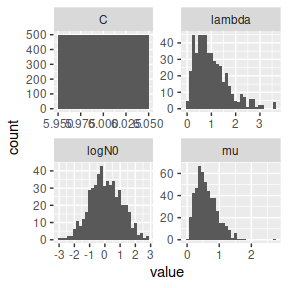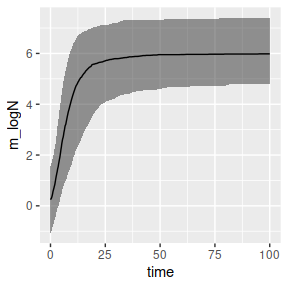# Custom distributions for uncertainty propagation

library(tidyverse)
library(biogrowth)

The biogrowth package includes two functions for making simulations including parameter uncertainty: predict_growth_uncertainty() and the generic predictMCMC(). The former includes parameter uncertainty considering that the model parameters follow a normal distribution with known mean and variance (defined on different scales). The latter, uses the Markov Chain of a model fitted using an MCMC algorithm to propagate the uncertainty in the parameter estimates.

Although these functions account for parameter uncertainty in the model predictions, they do not allow the definition of custom distribution for the model parameters. Nonetheless, that is relatively simple to do using the predict_growth() function together with the functions from the tidyverse.

First, we need to define the growth model to use and the time points where the solution is to be calculated. This is done in the same way as a “usual” calculation in predict_growth():

my_model <- "modGompertz"
my_time <- seq(0, 100, length = 1000) 

Next, we need to define the parameter sample. The tibble() function provides a convenient way for this. In this example, we will use 500 iterations, considering that $$\log N \sim Normal(0,1)$$, $$\mu \sim Gamma(3,5)$$ and $$\lambda \sim Gamma(2,2)$$ with $$C=6$$ constant.

set.seed(12412)
niter <- 500

par_sample <- tibble(logN0 = rnorm(niter, mean = 0, sd = 1),
C = 6,
mu = rgamma(niter, shape = 3, rate = 5),
lambda = rgamma(niter, shape = 2, rate = 2))

par_sample %>%
pivot_longer(everything()) %>%
ggplot() + geom_histogram(aes(value)) + facet_wrap("name", scales = "free")
#> stat_bin() using bins = 30. Pick better value with binwidth.The pmap() function from purrr provides a convenient way to convert the parameter sample to a list that defines the model and we can pass to predict_growth() using the map() function (also from purrr).

my_predictions <- par_sample %>%
pmap(., function(logN0, mu, lambda, C)
list(model = my_model,
logN0 = logN0,
mu = mu,
lambda = lambda,
C = C)
) %>%
map(.,
~ predict_growth(my_time, .)
)

Now, it is just a question of post-processing the simulations. For instance, we can calculate summary statistics of the simulations at each time point.

summary_preds <- my_predictions %>%
map(., ~.\$simulation) %>%
imap_dfr(., ~ mutate(.x, sim = .y)) %>%
group_by(time) %>%
summarize(m_logN = median(logN),
q10 = quantile(logN, .1),
q90 = quantile(logN, .9))

…that can be plotted using ggplot()

summary_preds %>%
ggplot(aes(x = time)) +
geom_ribbon(aes(ymin = q10, ymax = q90), alpha = .5) +
geom_line(aes(y = m_logN))